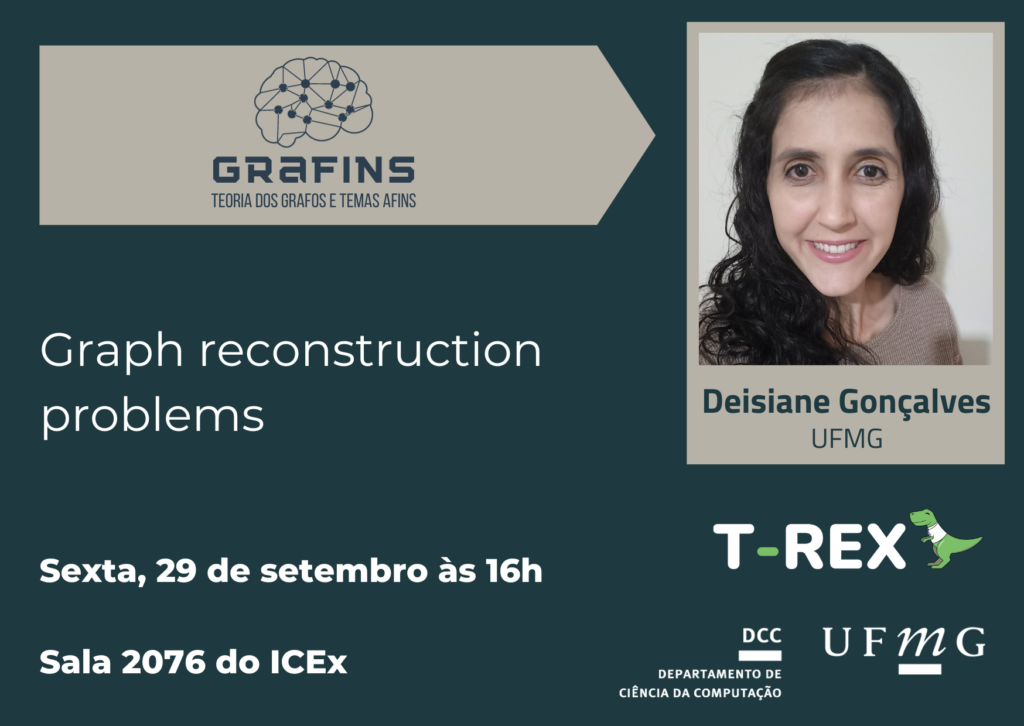# Palestra | Graph reconstruction problems | Deisiane Gonçalves, UFMG

Data e hora: sexta, 29 de setembro de 2023, às 16h.

Local: sala 2076 (ICEx) — atenção, fica ao lado da sala que tivemos os seminários anteriores.

Palestrante: Deisiane Lopes Gonçalves (UFMG)

Título: Graph reconstruction problems

Resumo: The vertex reconstruction conjecture asserts that every finite simple undirected graph on three or more vertices is determined, up to isomorphism, by its collection of unlabelled vertex-deleted subgraphs. The edge reconstruction conjecture asserts that every finite simple undirected graph with four or more edges is determined, up to isomorphism, by its collection of unlabelled edge-deleted subgraphs. We consider analogous problems of reconstructing an arbitrary graph G up to isomorphism from its abstract edge-subgraph poset, its abstract induced subgraph poset and its abstract bond lattice. We show that if a graph has no isolated vertices, then its abstract bond lattice and the abstract induced subgraph poset can be constructed from the abstract edge-subgraph poset except for the families of graphs that we characterise. The construction of the abstract induced subgraph poset from the abstract edge-subgraph poset generalises a well known result in reconstruction theory that states that the vertex deck of a graph with at least four edges and without isolated vertices can be constructed from its edge deck.

Given the vertex-deck of a graph G, all vertex-proper subgraphs of G can be counted using a basic result on graph reconstruction, known as Kelly’s lemma. We consider the problem of refining the lemma to count rooted subgraphs such that the root vertex coincides the deleted vertex. We show that such counting is not possible in general unless the vertex reconstruction conjecture is true, but a multiset of rooted subgraphs of a fixed height k can be constructed from the vertex-deck of G provided G has radius more than k. We prove analogous result for the edge reconstruction problem.

## Acesso por PERFIL

Pular para o conteúdo